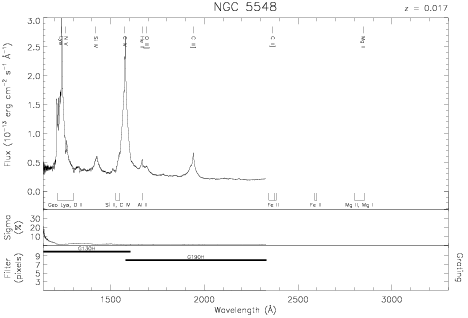Download dataset
TELESCOP= 'HST     '
INSTRUME= 'FOS     '
EQUINOX =               2000.0
DATE-OBS= '1993-04-19T11:23:36'
RA_PNT  =       214.4982041667
DEC_PNT =        25.1367555556
PA_PNT  =       310.3083456282
OBJECT  = 'NGC 5548'
ROOTNM01= 'Y1BP0104T'
GRNDMD01= 'SPECTROSCOPY'
DETECT01= 'BLUE    '
APERID01= 'A-1     '
FGWAID01= 'H19     '
ROOTNM02= 'Y1BP0105T'
GRNDMD02= 'SPECTROSCOPY'
DETECT02= 'BLUE    '
APERID02= 'A-1     '
FGWAID02= 'H13     '
ROOTNM03= 'Y1BP0204T'
GRNDMD03= 'SPECTROSCOPY'
DETECT03= 'BLUE    '
APERID03= 'A-1     '
FGWAID03= 'H19     '
ROOTNM04= 'Y1BP0205T'
GRNDMD04= 'SPECTROSCOPY'
DETECT04= 'BLUE    '
APERID04= 'A-1     '
FGWAID04= 'H13     '
ROOTNM05= 'Y1BP0304T'
GRNDMD05= 'SPECTROSCOPY'
DETECT05= 'BLUE    '
APERID05= 'A-1     '
FGWAID05= 'H19     '
ROOTNM06= 'Y1BP0305T'
GRNDMD06= 'SPECTROSCOPY'
DETECT06= 'BLUE    '
APERID06= 'A-1     '
FGWAID06= 'H13     '
ROOTNM07= 'Y1BP0404T'
GRNDMD07= 'SPECTROSCOPY'
DETECT07= 'BLUE    '
APERID07= 'A-1     '
FGWAID07= 'H19     '
ROOTNM08= 'Y1BP0405T'
GRNDMD08= 'SPECTROSCOPY'
DETECT08= 'BLUE    '
APERID08= 'A-1     '
FGWAID08= 'H13     '
ROOTNM09= 'Y1BP0504T'
GRNDMD09= 'SPECTROSCOPY'
DETECT09= 'BLUE    '
APERID09= 'A-1     '
FGWAID09= 'H19     '
ROOTNM10= 'Y1BP0505T'
GRNDMD10= 'SPECTROSCOPY'
DETECT10= 'BLUE    '
APERID10= 'A-1     '
FGWAID10= 'H13     '
ROOTNM11= 'Y1BP0604T'
GRNDMD11= 'SPECTROSCOPY'
DETECT11= 'BLUE    '
APERID11= 'A-1     '
FGWAID11= 'H19     '
ROOTNM12= 'Y1BP0605T'
GRNDMD12= 'SPECTROSCOPY'
DETECT12= 'BLUE    '
APERID12= 'A-1     '
FGWAID12= 'H13     '
ROOTNM13= 'Y1BP0704T'
GRNDMD13= 'SPECTROSCOPY'
DETECT13= 'BLUE    '
APERID13= 'A-1     '
FGWAID13= 'H19     '
ROOTNM14= 'Y1BP0705T'
GRNDMD14= 'SPECTROSCOPY'
DETECT14= 'BLUE    '
APERID14= 'A-1     '
FGWAID14= 'H13     '
ROOTNM15= 'Y1BP0804T'
GRNDMD15= 'SPECTROSCOPY'
DETECT15= 'BLUE    '
APERID15= 'A-1     '
FGWAID15= 'H19     '
ROOTNM16= 'Y1BP0805T'
GRNDMD16= 'SPECTROSCOPY'
DETECT16= 'BLUE    '
APERID16= 'A-1     '
FGWAID16= 'H13     '
ROOTNM17= 'Y1BP0904T'
GRNDMD17= 'SPECTROSCOPY'
DETECT17= 'BLUE    '
APERID17= 'A-1     '
FGWAID17= 'H19     '
ROOTNM18= 'Y1BP0905T'
GRNDMD18= 'SPECTROSCOPY'
DETECT18= 'BLUE    '
APERID18= 'A-1     '
FGWAID18= 'H13     '
ROOTNM19= 'Y1BP0A04T'
GRNDMD19= 'SPECTROSCOPY'
DETECT19= 'BLUE    '
APERID19= 'A-1     '
FGWAID19= 'H19     '
ROOTNM20= 'Y1BP0A05T'
GRNDMD20= 'SPECTROSCOPY'
DETECT20= 'BLUE    '
APERID20= 'A-1     '
FGWAID20= 'H13     '
ROOTNM21= 'Y1BP0B04M'
GRNDMD21= 'SPECTROSCOPY'
DETECT21= 'BLUE    '
APERID21= 'A-1     '
FGWAID21= 'H19     '
ROOTNM22= 'Y1BP0B05T'
GRNDMD22= 'SPECTROSCOPY'
DETECT22= 'BLUE    '
APERID22= 'A-1     '
FGWAID22= 'H13     '
ROOTNM23= 'Y1BP0C04T'
GRNDMD23= 'SPECTROSCOPY'
DETECT23= 'BLUE    '
APERID23= 'A-1     '
FGWAID23= 'H19     '
ROOTNM24= 'Y1BP0C05T'
GRNDMD24= 'SPECTROSCOPY'
DETECT24= 'BLUE    '
APERID24= 'A-1     '
FGWAID24= 'H13     '
ROOTNM25= 'Y1BP0D04T'
GRNDMD25= 'SPECTROSCOPY'
DETECT25= 'BLUE    '
APERID25= 'A-1     '
FGWAID25= 'H19     '
ROOTNM26= 'Y1BP0D05T'
GRNDMD26= 'SPECTROSCOPY'
DETECT26= 'BLUE    '
APERID26= 'A-1     '
FGWAID26= 'H13     '
ROOTNM27= 'Y1BP0E04T'
GRNDMD27= 'SPECTROSCOPY'
DETECT27= 'BLUE    '
APERID27= 'A-1     '
FGWAID27= 'H19     '
ROOTNM28= 'Y1BP0E05T'
GRNDMD28= 'SPECTROSCOPY'
DETECT28= 'BLUE    '
APERID28= 'A-1     '
FGWAID28= 'H13     '
ROOTNM29= 'Y1BP0F04T'
GRNDMD29= 'SPECTROSCOPY'
DETECT29= 'BLUE    '
APERID29= 'A-1     '
FGWAID29= 'H19     '
ROOTNM30= 'Y1BP0F05T'
GRNDMD30= 'SPECTROSCOPY'
DETECT30= 'BLUE    '
APERID30= 'A-1     '
FGWAID30= 'H13     '
ROOTNM31= 'Y1BP0G04T'
GRNDMD31= 'SPECTROSCOPY'
DETECT31= 'BLUE    '
APERID31= 'A-1     '
FGWAID31= 'H19     '
ROOTNM32= 'Y1BP0G05T'
GRNDMD32= 'SPECTROSCOPY'
DETECT32= 'BLUE    '
APERID32= 'A-1     '
FGWAID32= 'H13     '
ROOTNM33= 'Y1BP0H04T'
GRNDMD33= 'SPECTROSCOPY'
DETECT33= 'BLUE    '
APERID33= 'A-1     '
FGWAID33= 'H19     '
ROOTNM34= 'Y1BP0H05T'
GRNDMD34= 'SPECTROSCOPY'
DETECT34= 'BLUE    '
APERID34= 'A-1     '
FGWAID34= 'H13     '
ROOTNM35= 'Y1BP0I04T'
GRNDMD35= 'SPECTROSCOPY'
DETECT35= 'BLUE    '
APERID35= 'A-1     '
FGWAID35= 'H19     '
ROOTNM36= 'Y1BP0I05T'
GRNDMD36= 'SPECTROSCOPY'
DETECT36= 'BLUE    '
APERID36= 'A-1     '
FGWAID36= 'H13     '
ROOTNM37= 'Y1BP0J04T'
GRNDMD37= 'SPECTROSCOPY'
DETECT37= 'BLUE    '
APERID37= 'A-1     '
FGWAID37= 'H19     '
ROOTNM38= 'Y1BP0J05T'
GRNDMD38= 'SPECTROSCOPY'
DETECT38= 'BLUE    '
APERID38= 'A-1     '
FGWAID38= 'H13     '
ROOTNM39= 'Y1BP0K04T'
GRNDMD39= 'SPECTROSCOPY'
DETECT39= 'BLUE    '
APERID39= 'A-1     '
FGWAID39= 'H19     '
ROOTNM40= 'Y1BP0K05T'
GRNDMD40= 'SPECTROSCOPY'
DETECT40= 'BLUE    '
APERID40= 'A-1     '
FGWAID40= 'H13     '
ROOTNM41= 'Y1BP0L04T'
GRNDMD41= 'SPECTROSCOPY'
DETECT41= 'BLUE    '
APERID41= 'A-1     '
FGWAID41= 'H19     '
ROOTNM42= 'Y1BP0L05T'
GRNDMD42= 'SPECTROSCOPY'
DETECT42= 'BLUE    '
APERID42= 'A-1     '
FGWAID42= 'H13     '
ROOTNM43= 'Y1BP0M04T'
GRNDMD43= 'SPECTROSCOPY'
DETECT43= 'BLUE    '
APERID43= 'A-1     '
FGWAID43= 'H19     '
ROOTNM44= 'Y1BP0M05T'
GRNDMD44= 'SPECTROSCOPY'
DETECT44= 'BLUE    '
APERID44= 'A-1     '
FGWAID44= 'H13     '
ROOTNM45= 'Y1BP0N04T'
GRNDMD45= 'SPECTROSCOPY'
DETECT45= 'BLUE    '
APERID45= 'A-1     '
FGWAID45= 'H19     '
ROOTNM46= 'Y1BP0N05T'
GRNDMD46= 'SPECTROSCOPY'
DETECT46= 'BLUE    '
APERID46= 'A-1     '
FGWAID46= 'H13     '
ROOTNM47= 'Y1BP0O04T'
GRNDMD47= 'SPECTROSCOPY'
DETECT47= 'BLUE    '
APERID47= 'A-1     '
FGWAID47= 'H19     '
ROOTNM48= 'Y1BP0O05T'
GRNDMD48= 'SPECTROSCOPY'
DETECT48= 'BLUE    '
APERID48= 'A-1     '
FGWAID48= 'H13     '
ROOTNM49= 'Y1BP0P04T'
GRNDMD49= 'SPECTROSCOPY'
DETECT49= 'BLUE    '
APERID49= 'A-1     '
FGWAID49= 'H19     '
ROOTNM50= 'Y1BP0P05T'
GRNDMD50= 'SPECTROSCOPY'
DETECT50= 'BLUE    '
APERID50= 'A-1     '
FGWAID50= 'H13     '
ROOTNM51= 'Y1BP0Q04T'
GRNDMD51= 'SPECTROSCOPY'
DETECT51= 'BLUE    '
APERID51= 'A-1     '
FGWAID51= 'H19     '
ROOTNM52= 'Y1BP0Q05M'
GRNDMD52= 'SPECTROSCOPY'
DETECT52= 'BLUE    '
APERID52= 'A-1     '
FGWAID52= 'H13     '
ROOTNM53= 'Y1BP0R04T'
GRNDMD53= 'SPECTROSCOPY'
DETECT53= 'BLUE    '
APERID53= 'A-1     '
FGWAID53= 'H19     '
ROOTNM54= 'Y1BP0R05T'
GRNDMD54= 'SPECTROSCOPY'
DETECT54= 'BLUE    '
APERID54= 'A-1     '
FGWAID54= 'H13     '
ROOTNM55= 'Y1BP0S04T'
GRNDMD55= 'SPECTROSCOPY'
DETECT55= 'BLUE    '
APERID55= 'A-1     '
FGWAID55= 'H19     '
ROOTNM56= 'Y1BP0S05T'
GRNDMD56= 'SPECTROSCOPY'
DETECT56= 'BLUE    '
APERID56= 'A-1     '
FGWAID56= 'H13     '
ROOTNM57= 'Y1BP0T04T'
GRNDMD57= 'SPECTROSCOPY'
DETECT57= 'BLUE    '
APERID57= 'A-1     '
FGWAID57= 'H19     '
ROOTNM58= 'Y1BP0T05T'
GRNDMD58= 'SPECTROSCOPY'
DETECT58= 'BLUE    '
APERID58= 'A-1     '
FGWAID58= 'H13     '
ROOTNM59= 'Y1BP0U04T'
GRNDMD59= 'SPECTROSCOPY'
DETECT59= 'BLUE    '
APERID59= 'A-1     '
FGWAID59= 'H19     '
ROOTNM60= 'Y1BP0U05T'
GRNDMD60= 'SPECTROSCOPY'
DETECT60= 'BLUE    '
APERID60= 'A-1     '
FGWAID60= 'H13     '
ROOTNM61= 'Y1BP0V04T'
GRNDMD61= 'SPECTROSCOPY'
DETECT61= 'BLUE    '
APERID61= 'A-1     '
FGWAID61= 'H19     '
ROOTNM62= 'Y1BP0V05T'
GRNDMD62= 'SPECTROSCOPY'
DETECT62= 'BLUE    '
APERID62= 'A-1     '
FGWAID62= 'H13     '
ROOTNM63= 'Y1BP0W04T'
GRNDMD63= 'SPECTROSCOPY'
DETECT63= 'BLUE    '
APERID63= 'A-1     '
FGWAID63= 'H19     '
ROOTNM64= 'Y1BP0W05T'
GRNDMD64= 'SPECTROSCOPY'
DETECT64= 'BLUE    '
APERID64= 'A-1     '
FGWAID64= 'H13     '
ROOTNM65= 'Y1BP0X04T'
GRNDMD65= 'SPECTROSCOPY'
DETECT65= 'BLUE    '
APERID65= 'A-1     '
FGWAID65= 'H19     '
ROOTNM66= 'Y1BP0X05T'
GRNDMD66= 'SPECTROSCOPY'
DETECT66= 'BLUE    '
APERID66= 'A-1     '
FGWAID66= 'H13     '
ROOTNM67= 'Y1BP0Y04T'
GRNDMD67= 'SPECTROSCOPY'
DETECT67= 'BLUE    '
APERID67= 'A-1     '
FGWAID67= 'H19     '
ROOTNM68= 'Y1BP0Y05T'
GRNDMD68= 'SPECTROSCOPY'
DETECT68= 'BLUE    '
APERID68= 'A-1     '
FGWAID68= 'H13     '
ROOTNM69= 'Y1BP0Z04T'
GRNDMD69= 'SPECTROSCOPY'
DETECT69= 'BLUE    '
APERID69= 'A-1     '
FGWAID69= 'H19     '
ROOTNM70= 'Y1BP0Z05T'
GRNDMD70= 'SPECTROSCOPY'
DETECT70= 'BLUE    '
APERID70= 'A-1     '
FGWAID70= 'H13     '
ROOTNM71= 'Y1BP1004T'
GRNDMD71= 'SPECTROSCOPY'
DETECT71= 'BLUE    '
APERID71= 'A-1     '
FGWAID71= 'H19     '
ROOTNM72= 'Y1BP1005T'
GRNDMD72= 'SPECTROSCOPY'
DETECT72= 'BLUE    '
APERID72= 'A-1     '
FGWAID72= 'H13     '
ROOTNM73= 'Y1BP1104T'
GRNDMD73= 'SPECTROSCOPY'
DETECT73= 'BLUE    '
APERID73= 'A-1     '
FGWAID73= 'H19     '
ROOTNM74= 'Y1BP1105T'
GRNDMD74= 'SPECTROSCOPY'
DETECT74= 'BLUE    '
APERID74= 'A-1     '
FGWAID74= 'H13     '
ROOTNM75= 'Y1BP1204T'
GRNDMD75= 'SPECTROSCOPY'
DETECT75= 'BLUE    '
APERID75= 'A-1     '
FGWAID75= 'H19     '
ROOTNM76= 'Y1BP1205T'
GRNDMD76= 'SPECTROSCOPY'
DETECT76= 'BLUE    '
APERID76= 'A-1     '
FGWAID76= 'H13     '
ROOTNM77= 'Y1BP1304T'
GRNDMD77= 'SPECTROSCOPY'
DETECT77= 'BLUE    '
APERID77= 'A-1     '
FGWAID77= 'H19     '
ROOTNM78= 'Y1BP1305T'
GRNDMD78= 'SPECTROSCOPY'
DETECT78= 'BLUE    '
APERID78= 'A-1     '
FGWAID78= 'H13     '